# Applications of Double Integrals

Examples of applications of double integrals to calculate volumes and areas are presented along with their detailed solutions. 

## Calculate Volume Using Double

The volume $V$ of the solid that is above region $R$ in the $xy$-plane and below the surface $z = f(x,y)$, given that $f(x,y) \ge 0$, is given by the double integral  $\displaystyle V = \iint_R f(x,y) \;dy \;dx$

Example 1
Use the double integral to calculate the volume of the solid that that lies between the plane $z = 0$ and the paraboloid $z = 4 - x^2 - y^2$.
Solution to Example 1
The solid between the the plane $z = 0$ and the parabolid is shown below.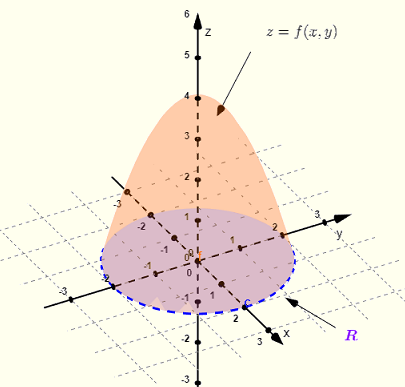Fig.1 - Volume Between the Plane $z = 0$ and the Paraboloid $z = 4 - x^2 - y^2$ The region $R$ of integration is the intersection of the paraboloid and the plane $z =0$ which is obtained by setting $z = 0$ in the equation of the paraboloid to obtain
$0 = 4 - x^2 - y^2$
Rewrite in standard form
$x^2 + y^2 = 2^2$
which is a circle on the $x y$ plane (or $z = 0$ ) with center at $(0,0)$ and a radius equal to $2$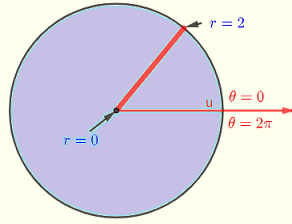Fig.2 - Region $R$ of integration which a circle with center at $(0,0)$ and a radius equal to $2$ Since the region of integration is a circle, it is more efficient to use double integrals in polar coordinates.
The volume $V$ is calculated using the double integral
$V = \displaystyle \iint_R f(x,y) \;dy \;dx$ , where $R$ is the region of integration defined by
$R: 0\le \theta \le 2\pi , 0 \le r \le 2$
which is the circle in Fig.2 and $f(x,y) = z = 4 - x^2 - y^2$
In polar coordinate, the volume is given by
$\displaystyle V = \int_0^{2\pi} \int_0^2 (4-r^2) r dr d\theta$
$\displaystyle V = \int_0^{2\pi} \int_0^2 (4-r^2) r dr d\theta$
$= \int_0^{2\pi} \left[2r^2-\frac{r^4}{4}\right]_0^2 d\theta$
$= \int_0^{2\pi} ( 4 ) d\theta$
$= 8 \pi$

Example 2
Find the constant $a$ (see diagram below) so that the volume of the solid that lies above the region $R$ located in the $x y$ plane shown below and the surface defined by the equation $z = \sqrt{1-x^2-y^2}$ is equal to $1$ cubic unit.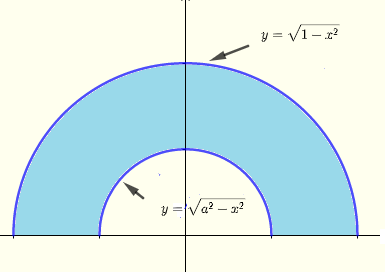Fig.3 - Given region of integration Solution to Example 2
We first convert the given region in Fig. 2 into polar coordinates. The outer half circle $y = \sqrt {1-x^2}$ has radius equal to $1$ and the inner $y = \sqrt {a^2-x^2}$ half circle has radius $a$ to be found.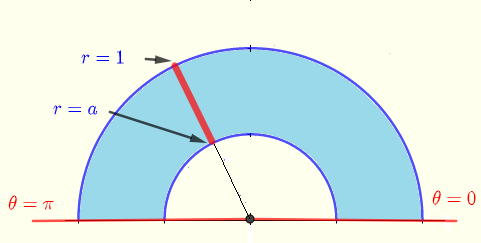Fig.4 - Region of integration in polar coordinates The region of integration in polar coordinates may be defined as
$R: 0\le \theta \le \pi , a \le r \le 1$
In poplar coordinates
$z = \sqrt{1-x^2-y^2} = \sqrt{1-r^2}$
In polar coordinate, the volume is given by
$\displaystyle V = \int_0^{\pi} \int_a^1 \sqrt{1-r^2} r dr d\theta$
$\displaystyle = \int_0^{\pi} \dfrac{\left(-a^2+1\right)^{\frac{3}{2}}}{3} d\theta$
$= \dfrac{\left(-a^2+1\right)^{\frac{3}{2}}}{3} \pi$
We want the volume $V$ to be equal to $1$, hence
$\dfrac{\left(-a^2+1\right)^{\frac{3}{2}}}{3} \pi = 1$
Solve the above for $a$
$a = \sqrt{1-\sqrt{\dfrac{9}{\pi ^2}}} \approx 0.174$

Example 3
Find $a$ so that the volume of the tetrahedron bounded by the planes $2x + 2 y + z = a , a \gt 0$, $y = 2 x$ , $y = 0$ and $z = 0$ is equal to $10$ cubic units.
Solution to Example 3
Below are graphed the planes that define the solid whose volume is to be calculated.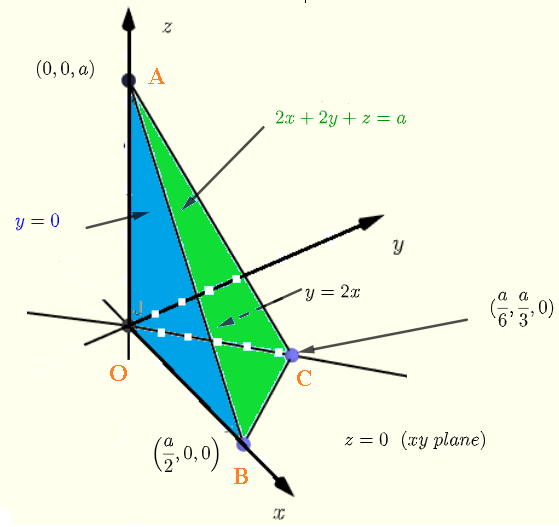Fig.5 - Planes that define the solid First find the region of integration which is a triangle defined by three vertices: $O , B$ and $C$
$O$ is the origin of the system of axis.
$B$ is the point of intersection and the $x$-axis and is found by setting $z = 0$ and $y = 0$ in the equation of the given plane $2x + 2 y + z = a$.
$2x = a$
Solve for $x$
$x = \dfrac{a}{2}$
$C$ is the point of intersection of the planes $2x + 2 y + z = a$ and $y = 2 x$ and is located on the $x y$ plane. A point on the $x y$ plane has $z = 0$.
Point $C$ is obtained by setting $z = 0$ in the equation $2x + 2 y + z = a$ solving the equation obtained
$2x + 2 y = a$ and $y = 2 x$
Solve the above by substitution to obtain
$y = a/3$ and $x = a/6$ Hence the region $R$ of integration on the $x y$ plane is a triangle with vertices
$O(0,0)$ , $B(a/2,0)$ and $C(a/6,a/3)$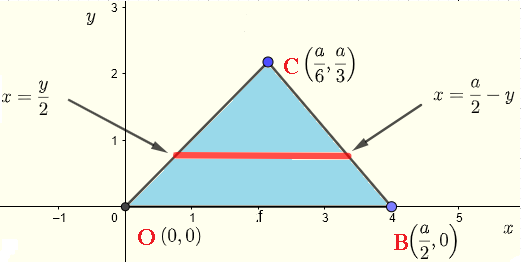Fig.6 - Region of integration
The volume $V$ of the tetrahedron is given by
$V = \displaystyle \iint_R f(x,y) \;dy \;dx$ , where $R$ is the region of integration defined by
$R: \dfrac{y}{2} \le x \le \ \dfrac{a}{2} - y , 0 \le y \le \dfrac{a}{3}$
and
$f(x,y) = z = a - 2x - 2 y$
$V = \displaystyle \int_0^{\frac{a}{3}} \int_{\frac{y}{2}}^{ \frac{a}{2} - y} (a - 2x - 2 y) \;dx \;dy$
Calculate the inner integral
$V = \displaystyle \int_0^{\frac{a}{3}} \left[ax-2yx-x^2\right]_{\frac{y}{2}}^{ \frac{a}{2} - y} \;dy$

$V = \displaystyle \int_0^{\frac{a}{3}} \left(\frac{9y^2-6ay+a^2}{4}\right) \;dy$
Evaluate the outer integral
$V = \left[ \frac{1}{4} \left(3y^3-3a y^2+a^2 y \right) \right]_0^{\frac{a}{3}}$
$V = \frac{a^3}{36}$
Note: You may check that the result above corresponds to the one that what may be obtained using the very well known formula for the volume of the tetrahedron
$V = \dfrac{1}{3} A \times H$
where $A$ is the area of the base and $H$ is the height from the base to the apex of the tetrahedron.
You may check this if you wish.
For the volume to be equal to $10$
$\frac{a^3}{36} = 10$
Solve for $a$
$a = \sqrt {360} \approx \:7.11378$

## Calculate Area of Surface Using Double

The area $A$ of a region $R$ in the plane $x y$ is given by the double integral  $A = \displaystyle \iint_R 1 \;dy \;dx$

Example 4
Use double integral to calculate the area of the region bounded by the curves $y = x^2$ and $y = - (x-2)^2 +4$
Solution to Example 4
We first plot the two curves and define the region bounded by the two curves.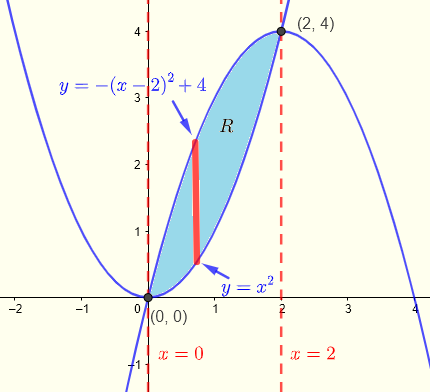Fig.7 - Region of integration The two curves intersect at the points that are solutions to the system of equations of the two curves
$y = x^2$ and $y = - (x-2)^2 +4$
which can be solved by substitution
$x^2 = - (x-2)^2 +4$
Expand and simplify
$2 x^2 - 4x = 0$
$2x(x-4) = 0$
The above equations has two solutions
$x = 0$ and $x = 4$
Use $y = x^2$ to calculate the $y$coordinate and hence the points
$(0,0)$ and $(2,4)$
The region $R$ enclosed by the two curves may be defined as follows
$R: 0 \le x \le 2 , x^2 \le y \le - (x-2)^2 +4$
The area $A$ is given by
$A = \displaystyle \int_0^2 \int_{x^2}^{- (x-2)^2 +4} 1 \;dy \;dx$
Calculate the inner integral
$A = \displaystyle \int_0^2 \left[y \right]_{x^2}^{- (x-2)^2 +4} \;dx$
Simplify
$A = \displaystyle \int_0^2 (-2x^2+4x) \;dx$
$A = \left[-\frac{2x^3}{3}+2x^2\right]_0^2 = 8/3$

Example 5
Use double integral to calculate the area of the region common to the two circles having equations $x^2 + (y-2)^2 = 9$ and $x^2 + y^2 = 9$
Solution to Example 5
We first plot the two curves and define the region bounded by the two curves. The region common to both circles is in light blue.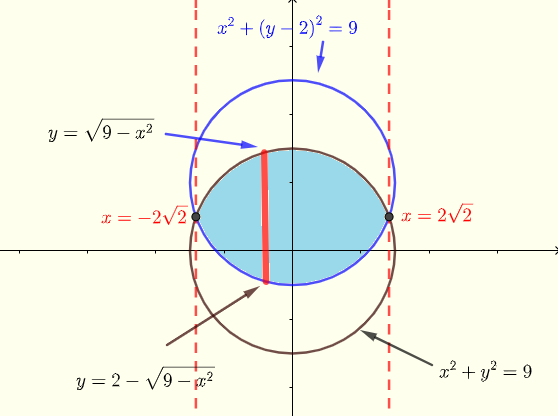Fig.7 - Common region to the two circles The points of intersection are found by solving the system of equations
$x^2 + (y-2)^2 = 9$ and $x^2 + y^2 = 9$
Expand the equation on the left
$x^2 + y^2 - 4 y + 4 = 9$
$x^2 + y^2 = 9$
Subtract the equations
$- 4 y + 4 = 0$
Solve for y
$y = 1$
Substitute $y$ by $1$ in any of the equations and solve for $x$ to obtain
$x = \pm 2 \sqrt 2$
We may now define region $R$ as
$R: -2\sqrt 2 \le x \le 2\sqrt 2 , 2 - \sqrt{9-x^2} \le y \le \sqrt{9-x^2}$
The area of the region $R$ is given by
$A = \displaystyle \int_{-2\sqrt 2}^{2\sqrt 2} \int_{2 - \sqrt{9-x^2}}^{\sqrt{9-x^2}} 1 \;dy \;dx$
Calculate the inner integral
$A = \displaystyle \int_{-2\sqrt 2}^{2\sqrt 2} \; \left[ y \right]_{2 - \sqrt{9-x^2}}^{\sqrt{9-x^2}} \; \;dx$
$A = \displaystyle \int_{-2\sqrt 2}^{2\sqrt 2} \left( 2 \sqrt{9-x^2} - 2 \right) \;dx$
$A = \left[ x\sqrt{9-x^2} + 9\arcsin(x/3) - 2x \right]_{-2\sqrt 2}^{2\sqrt 2}$
Note: that details of the integral $\int \sqrt{9-x^2}dx$ is included in appendix A.
Evaluate $A$
$A = 18\arcsin \left(\frac{2\sqrt{2}}{3}\right)-4\sqrt{2} \approx 16.5$

## Appendices

Appendix A
Calculate the integral
$\displaystyle I = \int \sqrt{9-x^2}dx$
Substitute
$x = 3 \sin u$ which gives $u = \arcsin (x/3)$ and $dx = 3 cos u \; du$
$9 - x^2 = 9 - 9 \sin^2 u$
$= 9(1-\sin^2) = 9 \cos^2 u$
and
$\sqrt{9-x^2} = \sqrt{9 \cos^2 u} = 3 \cos u$
Substitute and write
$\displaystyle I = \int \sqrt{9-x^2} \; dx = \int (3 \cos u ) \; 3 \cos u \; du$
$\displaystyle = 9 \int \cos^2 u du$
Use power reduction trigonometric identity to write $\cos^2 u = \dfrac{\cos(2u)+1}{2}$
$\displaystyle = \dfrac{9}{2} \int \left( \cos(2u)+1\right) du$
Evaluate
$\displaystyle I = \dfrac{9}{2} \left( \dfrac{\sin (2u)}{2} + u \right)$
Use the trigonomrtic identity $\sin (2u) = 2 \sin u \cos u$
$\displaystyle I = \dfrac{9}{2} \left( \sin u \cos u + u \right)$
Substitute $u$ by $\arcsin (x/3)$ , $\sin u = \dfrac{x}{3}$ , $\sin u = \sqrt {1-sin^2 u } = \sqrt {1 - x^2/9}$ to rewrite
$\displaystyle I = \dfrac{9}{2} \left( \dfrac{x}{3} \sqrt {1 - \dfrac{x^2}{9}} + \arcsin \left(\dfrac{x}{3}\right) \right)$
$\displaystyle I = \dfrac{x}{2} \sqrt {9 - x^2} + \dfrac{9}{2} \arcsin \left(\dfrac{x}{3} \right)$

## More Questions with Answers

Part 1
1. Find the volume of the solid bounded by the planes $x = 1$ , $x =2$ , $y = 0$ , $y = 2$ , $z = 0$ and lies under the hyperbolic paraboloid $z = 20 + x^2 - 2y^2$.
2. Find the volume of the solid bounded by the planes $y = 2$ , $z = 0$ and lies under the surface $z = 9-x^2$.
3. Find the volume of the solid bounded by the cylinder $9 - x^2 - y^2 = 0$ , $z = 0$ and lies under the plane $z=5+0.5x+y$.
4. Find area of the region in the $x y$ plane enclosed by the circle of equation $x^2 + y^2 = 4$ and the line $y = - 1$.

## Answers to the Above Questions

Part 1:
1. $\dfrac{118}{3}$
2. $72$
3. $45 \pi$
4. $\dfrac{8\pi }{3}+\sqrt{3}$

## More References and Links

1. Double Integrals Calculations
2. Double Integrals Over General Regions
3. Double Integrals in Polar Coordinates
4. Howard Anton, Irl C. Bivens, Stephen Davis ; Calculus: Early Transcendentals; Willey, 2012.
5. Gilbert Strang; MIT, Calculus, Wellesley-Cambridge Press, 1991
6. Joel Hass, University of California, Davis; Maurice D. Weir Naval Postgraduate School; George B. Thomas, Jr.Massachusetts Institute of Technology ; University Calculus , Early Transcendentals, Third Edition , Boston Columbus , 2016, Pearson.
Engineering Mathematics with Examples and Solutions